• 自相关函数R(t1,t2)：为了衡量随机过程x(t)在任意两个时刻(t1,t2)上获得随机变量之间关联程度。 R(t1,t2) = E[ x(t1) x(t2) ] 或者写成 R(τ) = E[ x(t) x(t+τ) ] 互相关函数：是描述随机信号x(t),y(t)在...
首先，概念解释:

自相关函数R(t1,t2)：为了衡量随机过程x(t)在任意两个时刻(t1,t2)上获得的随机变量之间的关联程度。

                                    R(t1,t2) = E[ x(t1) x(t2) ]    或者写成 R(τ) = E[ x(t) x(t+τ) ] 

互相关函数：是描述随机信号x(t),y(t)在任意两个不同时刻t1，t2的取值之间的相关程度。：是描述随机信号x(t),y(t)在任意两个不同时刻t1，t2的取值之间的相关程度。

R(t1,t2) = E[ x(t1) y(t2) ]   

平稳随机过程：是一类应用非常广泛的随机过程，统计特性和时间起点无关。均值与t无关，为常数a；自相关函数只和时间间隔

τ有关， R(t1,t1+τ) =R(τ) 。平稳过程的功率谱密度与其自相关函数是一对傅里叶变化（Winner-Khintchine定理）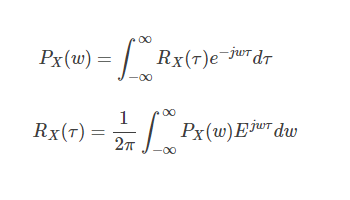在信号处理领域，自相关和互相关函数的定义如下：

设原函数是f(t)，则自相关函数定义为R(u)=f(t)*f(-t)，其中*表示卷积；

设两个函数分别是f(t)和g(t)，则互相关函数定义为R(u)=f(t)*g(-t)，它反映的是两个函数在不同的相对位置上互相匹配的程度。

 

从数学的角度来讲,相关是一个与卷积类似的运算.相关是指将一个函数滑过另一个函数并求出两者乘积下的面积.在卷积的运算中,其中一个函数要针对纵轴做翻转,然后再求两函数滑动相乘的面积和.而相关运算中,两个函数不做任何翻转直接进行相对滑动的乘积面积和.

进而,两个相同函数的相关运算称为自相关.而两个不相同的函数的相关运算称为互相关.

那么,其实从定义中我们很好理解.一般来讲,自相关函数得到的自相关运算是比较大的.因为两个相同的信号相互滑过,相乘的面积肯定是很大的.但当两个函数的相关性为0的时候,两个函数就不会有重合的情况,在时域上来讲就不可能存在相似的情况

通过这个解释，让我更好的理解了“相关”的概念。

展开全文• 有限长信号自相关函数的估计对于广义平稳随机信号的自相关函数有计算公式如下：而在实际中，我们遇到的物理信号一般为因果性的实信号，此时，自相关函数的表示可以简化为：当信号在有限点采样时，我们只能获得自相关...

有限长信号自相关函数的估计

对于广义平稳随机信号的自相关函数有计算公式如下：而在实际中，我们遇到的物理信号一般为因果性的实信号，此时，自相关函数的表示可以简化为：当信号在有限点采样时，我们只能获得自相关函数的一个估计值，常用的估计方法包括：直接估计（根据定义计算）和能

量谱估计（快速算法）。

自相关函数的直接估计

对于长度为N的有限长信号，自相关函数的计算公式为：由于信号只有N-1个值，因此对于每个延迟m，可以利用的数据只有N-1-m个，因此实际计算时，可写为：该方法是自相关函数的有偏一致估计。自相关函数的无偏估计为：但是无偏估计的方差性能不好，不是一致估计，因此较少使用。

由傅里叶变换计算自相关函数

由有限长信号自相关函数的定义可得：对其进行傅里叶变换：将序列补零延拓为长度2N-1的序列，并记的傅里叶变换为，则上式变换为：因此可得结论：自相关函数与功率谱是一对傅里叶变换。并可得其一般步骤为：
1.       对信号补零，并进行DFT；
2.       求功率谱密度；

3.       进行IDFT，获得自相关函数；

从计算过程中可以看出，上述方法求得的是自相关函数的有偏一致估计。

计算实例及MATLAB代码

设有限长信号，计算其自相关函数。

1. 手工计算

将数据代入自相关函数的计算式：可得2. 用MATLAB进行直接估计

N = length(xn);
xn = [1, -1, 3, 2];
rm = xcorr(xn)/N;
rm的计算值为 [0.5, 0.25, 0.5, 3.75, 0.5, 0.25, 0.5]

3. 用DFT计算
N = length(xn);
p = fft(xn, 2*N-1);
pp = p.*conj(p);
rm = fftshift(ifft(pp)/N);
rm = [0.5, 0.25, 0.5, 3.75, 0.5, 0.25, 0.5]


展开全文matlab fft 算法
• 对于***平稳随机信号***相关函数的性质： Rxx(m)=E[Xn∗Xn+m]=E[XnXn−m∗]R_{xx}(m) = E[X_n^*X_{n + m}] = E[{X_n}X_{n - m}^*]Rxx​(m)=E[Xn∗​Xn+m​]=E[Xn​Xn−m∗​] Rxx(m)=Rxx(−m)R_{xx}(m) = R_{xx}( - ...
相关函数贯穿整个随机信号处理，下面就着重讨论相关函数。
自相关函数的定义
集合平均下自相关函数计算公式：
$R_{x x}(m, n)=E\left[X^{*}(m) X(n)\right]=\lim _{N \rightarrow \infty} \frac{1}{N} \sum_{i=1}^{N} x_{i}^{*}(m) x_{i}(n)$
时间平均下自相关函数的计算公式：
$< {x^*}(i)x(i + j) > = \mathop {\lim }\limits_{N \to \infty } {1 \over {2N + 1}}\sum\limits_{i = - N}^N {{x^*}(i)x(i + j)}$
平稳随机序列的三种定义不同写法：
(1) $r_{xx}(m) = E[x(n)x^*(n+m)], r_{xy}(m) = E[x(n)y^*(n+m)]$
(2) $r_{xx}(m) = E[x(n)x^*(n-m)], r_{xy}(m) = E[x(n)y^*(n-m)]$
(3) $r_{xx}(m) = E[x^*(n)x(n+m)], r_{xy}(m) = E[x^*(n)y(n+m)]$
三种不同写法的区别：
对于实信号，三者一致；
对于虚信号，为方便比较，令(2)中的$r_{xx}(m)$表示为${r}'_{xx}(m)$, 令(3)中的$r_{xx}(m)$表示为${r}''_{xx}(m)$
$r_{xx}(m) = {r}'_{xx}(-m)={r}''_{xx}(-m)$
平稳随机信号相关函数的性质
(1) $R_{xx}(m) = E[X_n^*X_{n + m}] = E[{X_n}X_{n - m}^*]$
(2) 自相关函数是偶函数
$R_{xx}(m) = R_{xx}( - m), R_{xy}^*(m) = {R_{yx}}( - m)$
(4) ${R_{xy}}(m) = {R_{xy}}(n,n - m) = E[X_n^*Y_{_{n + m}}^{}]$
(5)  $R_{xy}(m) =0$，两个序列正交
(6) $Rxx(0)$数值上等于随机序列的平均功率;（由定义可以直接求得）
(7) 相关性随时间差的增大越来越弱：
$\lim _{m \rightarrow \infty} R_{x x}(m)=\mu_{x}^{2} \quad \lim _{m \rightarrow \infty} R_{x y}(m)=\mu_{x} \mu_{y}$
相关函数的傅里叶变换和$Z$变换
对于非周期信号，能量时无穷的，无法使用傅里叶变化，但是信号的自相关函数可能存在傅里叶变换。由上述性质(7) 可知，当相关函数随着时间差趋于无穷时，自相关函数取值趋于均值的平方。当均值为0时，自相关函数就能收敛，傅里叶变换存在。
可以通过预处理，去均值，使自相关函数的均值为0。


展开全文• 随机信号的产生及期望计算，其样本函数 自相关函数 功率谱密度的显示，随机信号的平稳性分析
• 自协方差函数自协方差函数是描述随机信号 在任意两个不同时刻t，t-k，的取值之间的二阶混合中心矩，用来描述 在两个时刻取值的起伏变化（相对与均值）的相关程度，也称为中心化的自相关函数。协方差定义...
1. 样本自协方差函数2. 自协方差函数3. 自相关函数4. 偏自相关系数1. 样本自协方差函数对于满足均值遍历性、二阶矩遍历性的平稳时间序列一次具体观测值，总体平均可转化为时间平均，因此可计算：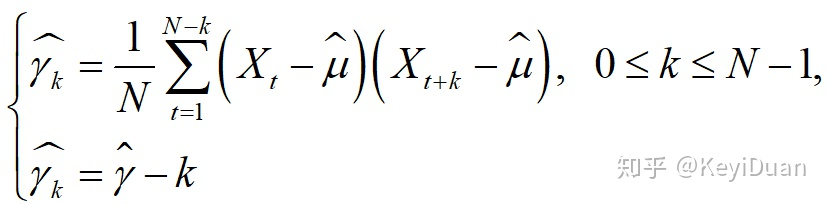2. 自协方差函数自协方差函数是描述随机信号在任意两个不同时刻t，t-k，的取值之间的二阶混合中心矩，用来描述在两个时刻取值的起伏变化（相对与均值）的相关程度，也称为中心化的自相关函数。协方差定义为：时间序列的自协方差指：时间序列或者信号，经过时间平移后，与自己的协方差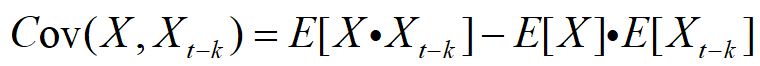而对于中心化AR模型，均值为0，即所以上式表示为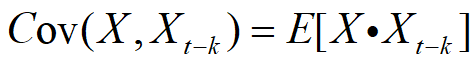在平稳AR模型等式两边同乘以，再取数学期望可得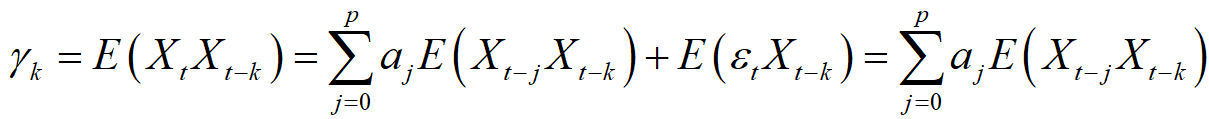得到自协方差函数的递推公式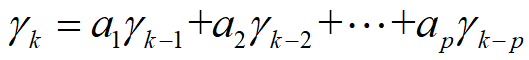Yule-Walker方程平稳AR序列的自协方差函数满足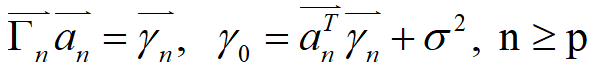其中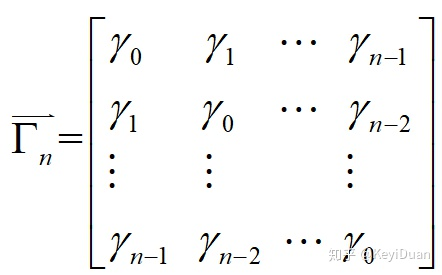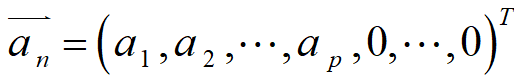此方程的意义在于：已知AR模型的具体形式，可计算出自协方差函数；已知自协方差函数，可以估计出模型的未知参数。而在实际应用中，选用样本自协方差函数估计自协方差函数3．自相关函数(Autocorrelation function)自相关函数是描述随机信号在任意两个不同时刻t，t+k的取值之间的相关程度公式定义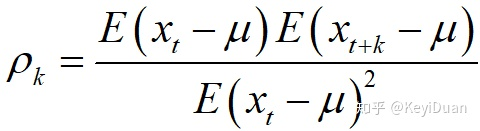对于零均值的平稳AR序列，自相关函数为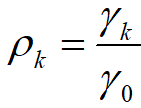自相关系数的递推公式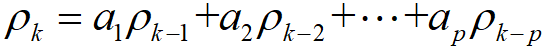自相关系数按负指数衰减且具有拖尾性4 . 偏自相关系数自相关系数直接反映了随机变量与之间的相关关系，不考虑中间k-1个随机变量的影响而偏自相关系数把中间k-1个随机变量看作已知的条件下来研究与之间的相关关系公式定义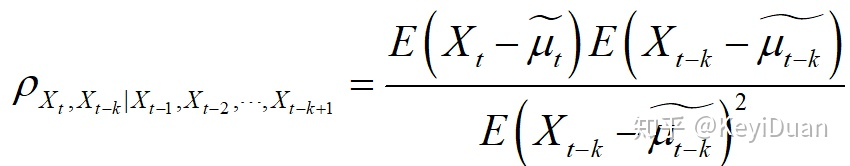其中，为条件期望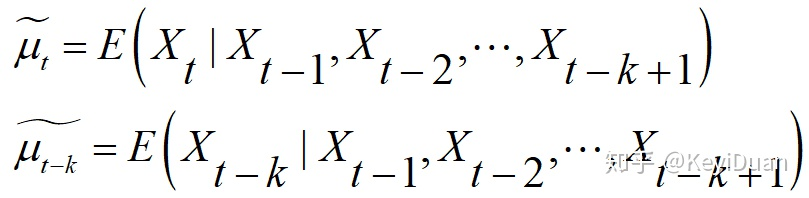考虑中心化平稳AR序列，可用过去的k个序列作k阶回归拟合,即：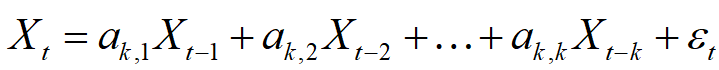两边取数学期望得：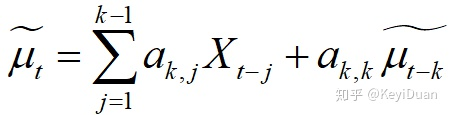则有：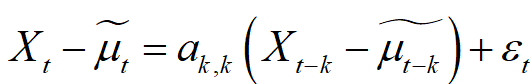所以， 偏自相关系数恰好就等于k阶自回归拟合中的滞后k阶偏自相关系数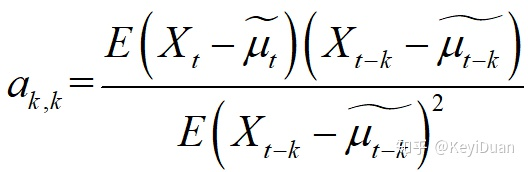也就是说，计算偏自相关系数可用通过计算k阶自回归拟合得到Levinson递推公式： 如果正定，对有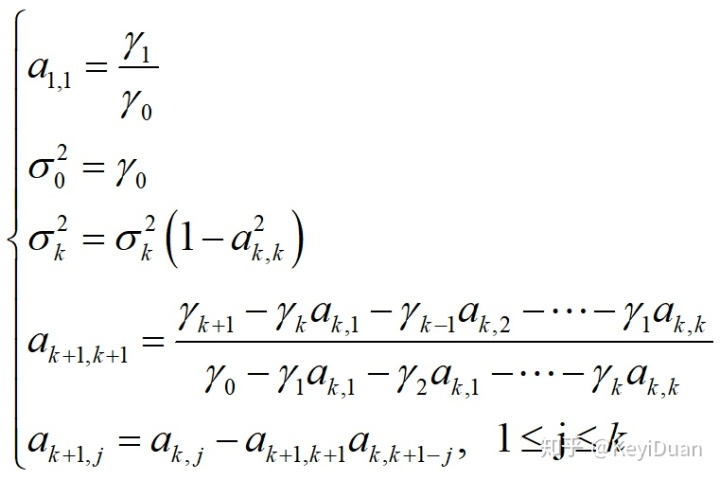偏自相关系数在p之后的值均为0 ，即截尾性
展开全文方差递推公式
• 来源：声振测试微信公众号，作者：于...设x(t) 为某点输入的平稳随机信号，y(t) 为任一点响应，也是随机平稳信号，则振动系统频率响应函数的互功率谱密度函数除以功率谱密度函数的商，即：式中，Sxx(f) 和Sxy(...xcorr函数
• 与马尔可夫链不同，有很多随机过程不仅与当前状态有关系，而且与...平稳随机过程有着非常重要数字特征，它均值函数为一个常数，并且自相关函数为事件差单值函数不随时间改变而改变。就是说随机过程中不...
• ①实平稳过程 的自相关函数是偶函数 ② ③非周期平稳过程 的自相关函数满足 （7）功率谱密度 已知随机信号 的功率谱 ，求其自相关函数与均方值。 （8）高斯过程和白噪声 ①随机过程的任意n维随机变量，服从...
• 文章目录随机信号的参数建模法MA模型AR模型ARMA模型AR模型参数的估计AR模型参数和自相关函数的关系实例Y-W方程的解法——L-D算法L-D算法的优缺点 随机信号的参数建模法 在对语音信号进行编码时，对语言产生的物理...
• 原理 对于确定性信号，可以用FFT做频域分析，得到其频域特性。对于平稳随机信号，因为是无限能量的信号，故其傅里叶...平稳随机信号的自相关函数的定义为： 式中，x(n)和y(n)都是平稳随机信号，“*”代表取共轭。如python matlab
• 2.随机信号功率谱估计方法：对于平稳随机信号，其功率谱与其自相关函数为傅里叶变换对。对于本例而言，其均值E[x(n)]为0，自相关函数R(m)与时间起点无关，只与时间间隔m有关因而具有平稳性，可以直接运用维纳-欣钦...
• 随机过程可以作为离散时间信号的模型。 通常，一个随机过程是一族带有序号的随机变量： ..., x[-2], x[-1], x, x, x, ... 上面的每一个x[i]都是一个随机变量，可以分别具有不同的概率分布（连续的或离散...
• 编写一个产生协方差函数为的平稳高斯过程程序，产生若干样本函数。估计所产生样本时间自相关函数和功率谱密度，并求统计自相关函数和功率谱密度，最后将结果与理论值比较。matlab
• 随机信号及其统计描述 随机过程统计描述 概率分布 数字特征 数学期望 表示所有样本在任意时刻t取值分布中心。 方差 样本相对于数学期望平均偏离程度 相关函数 自相关 同一随机过程在任意两个不同...
• Wiener 过程通过稳定连续线性系统后为平稳随机过程, 且均值和自相关函数阵为时间遍历。基于 状态空间分析,给出了连续随机信号建模时间序列分析方法,并证明了参数估计一致收敛性。仿真 结果显示了...时间序列分析 系统辨识
• AR模型参数估计 一、AR模型 ...二、AR模型参数和自相关函数的关系 1、推导过程 2、举例 已知自回归信号模型 **AR（3）**为： 式中 w( n ) 是具有方差 σ2 =1 的平稳白噪声，求 a. 求自相关序列
• 随机信号原理分析 随机信号的古典法谱估计 广义平稳随机过程的功率谱是自相关函数的福利叶变换它取决于无数个自相关函数值但对于许多实际问题可以利用的数据往往是有限的所以要准确的计算功率谱是不可能的比较合理的...
• ① 广义平稳随机信号的自相关函数和功率谱密度之间互为傅立叶变换关系 ② 计算基带信号的自相关函数 ③ 基带信号的均值和自相关函数是周期函数，因此数字基带信号不是广义平稳随机过程 ④ 对基带信号自相关函数在一...
• 第三：自相关函数RXR_XRX​也应该为等值序列 其中上面三个，哪个是首要，第一个需要求出？ 是均方值！为什么？ 因为均方值是包括直流分量、交流分量，它是总功率等于方差加上均值平方。 如果均方值相等，是否...
• 在下文中，你将会看到：平稳过程究竟有什么意义、随机信号处理是怎样与固定信号分析联系起来自相关函数的定义、自相关矩阵意义和计算 平稳过程 平稳过程是现代数字信号处理一个大问题 它定义是: 统计...
• 滚动轴承在工作过程中产生振动...用循环自相关函数谱图与包络频谱图进行对比分析,说明在提取滚动轴承故障特征时,利用循环自相关函数法能够很好地抑制噪声。所提出方法对于滚动轴承故障精细诊断具有重要意义。
• （2）维纳滤波要求过程的自相关系数和互相关函数的简单知识，而卡尔曼滤波则要求时域中状态变量及信号产生过程的详细知识；（3）维纳滤波要求平稳，而卡尔曼滤波则不要求。 1.1维纳滤波器原理 维纳滤波的原理是...matlab 卡尔曼滤波算法
• 随 机 序 列 功 率 谱 对于平稳随机序列X(n)，如果相关函数满足 ∑m=−∞+∞∣RX(m)∣<...那么它功率谱定义为自相关函数RX(m)R_X(m)RX​(m)傅里叶变换： GX(ejω)=∑m=−∞+∞∣RX(m)∣e−jmωG_X(e^{j\o...卷积 python
• 以无线光通信中的副载波调制技术为背景，研究了光强调制正交相移键控信号的循环自相关和循环谱密度函数，并对实际测得的信号的循环谱和循环自相关函数进行了分析。结果表明，无线光副载波信号经过大气传输，同时受到...
• 因为第二章确知信号主要是讲频域中功率信号、能量信号和功率信号的功率谱密度，能量信号的能量谱密度，时域中能量信号和功率信号的自相关函数和和互相关函数，在其他科目中会有讲解，所以这里不再多说。第三章将随机...
• 二阶统计量：自相关函数，互相关函数，自协方差函数，互协方差函数 2.解释随机过程平稳性与遍历性 答：平稳性：一个随机过程统计性质不随时间改变，就称为严平稳或强平稳或狭义平稳随机过程；一个随机过程...算法
• 随机过程的基本概念，统计特性—数字特征，平稳随机过程及其数字特征，平稳过程的自相关函数性质，各态，历经过程，两个随机过程的联合统计特性，随机过程的微分和积分，高斯随机过程，平稳过程的自功率谱分析，自...
• 注：本博客是基于奥本海姆《离散时间信号处理》第三版编写，主要是为了自己学习...为了分析过采样在该系统中效果，考虑一个零均值广义平稳的随机过程xa(t)，其功率谱密度记为，自相关函数记为。为了简化讨论，最...